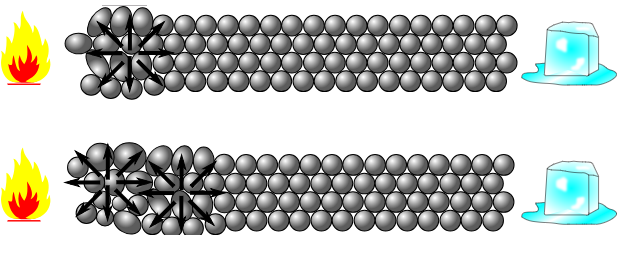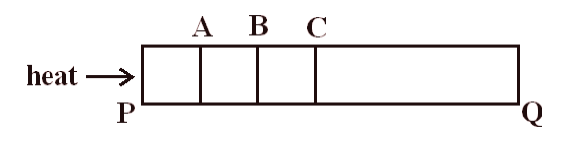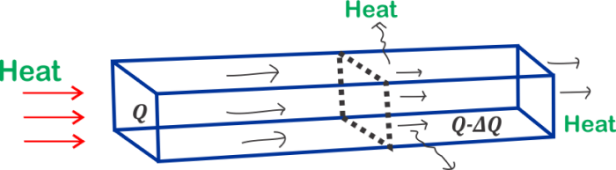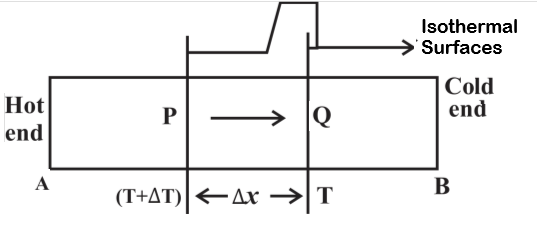# Heat Conduction

## What is conduction?

Heat conduction is the transfer of heat from the hotter part of the material to its colder part without the actual movement of the particles.
During the heat conduction process heat energy is transferred only to the successive molecules. In this process, molecules do not leave their mean position at all. So in conduction particles of the medium transfers heat without leaving the mean position.
Heat conduction does not take place in liquids and solids. This happens because molecules of both gases and liquids move randomly or in a specific direction.

## How do conduction heat transfer works

When one end of a metal bar is heated, molecules at this end begin to vibrate faster and faster. These particles at the hot end have a greater kinetic energy of vibration in compassion to the particles that are not directly under heat exposure. These faster moving particles/molecules collide with their neighboring molecules.Figure 3: Transfer of heat at molecular level. Here from the figure you can see how heat is being transferred at the molecular level through the vibrations of molecules at heated end to the colder end. During the collision, they transfer some of their energy to the neighboring molecules. This way faster moving or vibrating molecules transmit the heat energy to neighboring molecules. These, in turn, transfer some of their energy to the molecules near them. Thus the energy is transferred by molecular collision from the hot end of the object to its cold end. The transfer of heat continuous until two ends of the object are at the same temperature. This way energy of thermal motion is passed along from one molecule to the next keeping their mean position fixed.
Solid substances which conduct heat easily are good conductors of heat. For example silver, copper etc. All metals are a good conductor of heat.
Those substances which do not conduct heat easily are called bad conductors of heat. For examples of wood, cloth, air, paper etc. Generally, good conductors of heat are also good conductors of electricity.

## Everyday conduction examples

• In winter, metallic handle appears to be colder than the wooden door. This happens because metallic handle is the good conductor of heat and heat flows from our body to the handle. So it feels cold
• Cooking utensils are provided with wooden handles. This is because wooden handle do not allow conduction of heat from hot utensil to hand.
• A new quilt is warmer than old quilt because new quilt contains more air in its pores as compared to the old quilt.
• A refrigerator is provided with insulated walls to minimise the chance of heat flowing into the refrigerator from outside.

## Important terms related to Conduction

### (1) The steady state of a substance:-

To understand the steady state of a substance let us consider a solid metal bar. We now heat one end of the metal bar. This way one end of the rod is at a higher temperature and another end of the rod is at a lower temperature.From the discussion about the transfer of heat by conduction, we know that heat energy is transferred only to the successive molecules. Let us divide the solid metal bar into various segments as shown below in the figureFigure 4 : Transient or non stationary state of Heat Transfer through the process of conduction in a metal bar. If we start providing heat to the bar at end P then each section of the bar get heat from its adjacent previous section. That is section C will get heat from section B and section B will get heat from Section A.
Again consider sections A, B and section C. Now section B gets heat from section A. Some part of heat received is absorbed due to which temperature of section B increases. Some part of this heat received flows to the atmosphere. Remaining part of the heat is transferred to adjacent section C.
In this state temperature of every cross-section of the bar. Goes on increasing with time. This is the transient or variable state of heat conduction.Figure 5 : Transient or non stationary state of Heat Transfer through the process of conduction in a metal bar. With the passage of time and continuous supply of heat, a state is reached when the temperature of each section becomes uniform. In this state, no heat is absorbed by any section of the bar.
This state of the bar when the temperature of every cross-section of the bar becomes constant and there is no further absorption of heat in any part is called Steady-State or Stationery-State.
It is important to note here that
(1) The different sections of the metal bar have a different temperature, but the temperature of each section remains constant with the passage of time.
(2) The temperature decreases as me more away from the hot end. Heat lost to the atmosphere can be prevented by covering the metal bar with an insulating material. In this case, at a steady state total heat transfer take place by conduction. From hot and (higher temperature) of the bar to cold end (lower temperature).

### (2) Isothermal Surface :-

The surface of a substance whose all particles or molecules are at the same temperature is called an isothermal surface.
Figure 5 below shows two isothermal surface $P$ and $Q$. Here temperature of all points on surface $P$ is same. Temperature of surface $P$ is not same as the temperature of surface $Q$.

The rate of change of temperature with distance in the direction of flow of heat is called temperature gradient
Let us consider the figure given belowFigure 6 : Locus of equal temperatures form an isothermal surface.. Sections $P$ and $Q$ in the above figure are isothermal surfaces. Let $\Delta x$ be the normal distance between these two surfaces. Now the temperature of section $P$ is greater than the temperature of section $Q$. Let $(T+\Delta T)$ be the temperature of section $P$ and $T$ be the temperature of section $Q$; then the temperature gradient is written as
\begin{align} \text{grad.} T & = \frac{\text{change in Temp}}{\text{Normal distance}} \\ & =\frac{T-(T+\Delta T)}{\Delta x} \\ & =-\frac{\Delta T}{\Delta x} \\ \end{align} The temperature gradient is the positive quantity in direction of increasing temperature. Since heat always flows from higher temperature to lower temperature. The temperature gradient along the direction of heat flow has a negative value.

### (4) The rate of heat flow :-

The quantity of heat transferred from an isothermal region per unit time in the direction of heat propagation is called the rate of heat flow. It is denoted by $H$. It is also known as heat current.
If $Q$ is the amount of heat transferred in time t; then
$$H=\frac{Q}{T}$$ Units are $Kcal{s ^{ - 1}}$ , $J{s ^{ - 1}}$ and $cal{s ^{ - 1}}$

### (5) The specific rate of heat flow:-

The rate of heat flow per unit area is called the specific rate of heat flow. It is denoted by $q$. $q=\frac{H}{A}$
Units are $Kcal.{m^{ - 2}}{s^{ - 1}}$, $K{m^{ - 2}}{s^{ - 1}}$ and $Cal.cm^{ - 2}{s^{ - 1}}$